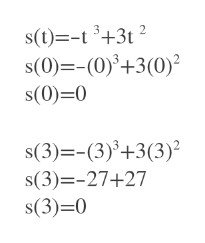Hello,this problem involves solving displacement and velocity given an equation...given s = f(t) of a body moving on a coordinate line, with s in meters and t in seconds... s = -t^3 + 3t^2, 0 <= t <= 3.  I am supposed to find the displacement and average velocity for the given time interval. I cant write the delta symbol on here, so i'll just spell it out on the function... the average velocity is = to the displacement/travel time.  and the formula for it is [f(t+DELTAt) - f(t)]/DELTAt     i am not sure what the DELTAt value would be, or how to get it. If you could explain that, and also show what you plugged in where in the formula, that would be great! Also (for instantaneous velocity) the equation is the same except it has a limit as DELTAt approaches 0, would you just plug in 0 for DELTAt in that case?thank you so much

Question

Hello,

this problem involves solving displacement and velocity given an equation...

given s = f(t) of a body moving on a coordinate line, with s in meters and t in seconds... s = -t^3 + 3t^2, 0 <= t <= 3.  I am supposed to find the displacement and average velocity for the given time interval. I cant write the delta symbol on here, so i'll just spell it out on the function... the average velocity is = to the displacement/travel time.  and the formula for it is

[f(t+DELTAt) - f(t)]/DELTAt     i am not sure what the DELTAt value would be, or how to get it. If you could explain that, and also show what you plugged in where in the formula, that would be great! Also (for instantaneous velocity) the equation is the same except it has a limit as DELTAt approaches 0, would you just plug in 0 for DELTAt in that case?

thank you so much

Step 1

We first find the distance travelled at t=0 and t=3

At t=0 it was at s=0

At t=3 also it is at s=0help_outlineImage Transcriptioncloses(t)-t3+3t2 s(0)=-(0)+3(0) s(0) 0 s(3)-(3)+3(3) s(3)-27+27 s(3)-0 fullscreen
Step 2

So displacement from t=0 to t=3 is s(3)-s(0)=0-0=0

Want to see the full answer?

See Solution

Want to see this answer and more?

Our solutions are written by experts, many with advanced degrees, and available 24/7

See Solution
Tagged in

Calculus